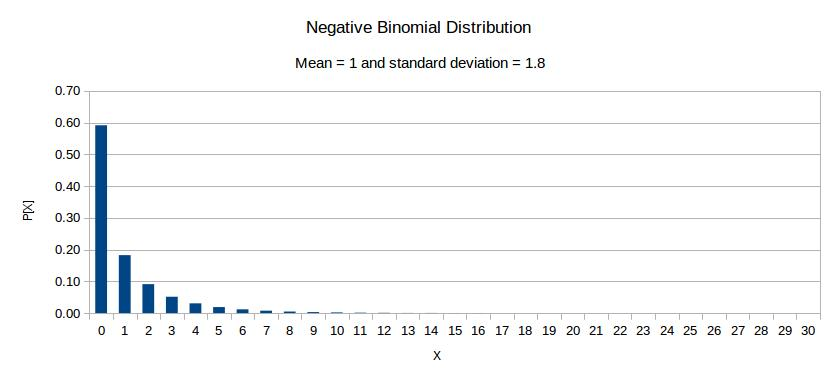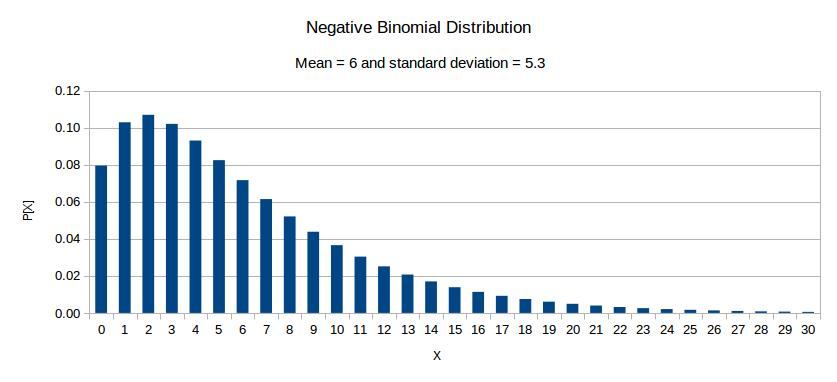# Monte Carlo Simulator – Part 2

This article contains detailed information concerning the online Monte Carlo inventory management simulator which can be accessed by clicking on the “Simulator” tab in the menu at the top of the page. In parts of this article, it will be assumed that the reader has some knowledge of statistics. If you don’t have a statistical background then you should still be able to understand the parts of the article which matter to you. Just ignore any sentence which contains terms which you don’t understand.

The simulations are carried out on a daily basis and continue until there have been at least 1000 customer demands.

In the event of a shortage, customer backordering takes place. I intend to allow for lost sales at a later date.

The daily customer demands are assumed to be independent and identically distributed negative binomial random variates. That type of distribution is a realistic one for modeling daily demands. It is a type of stuttering Poisson distribution. In other words, the daily number of customer requests is treated as having a Poisson distribution and the quantity requested by each customer can vary. The Poisson distribution is a special case. For items which move fairly fast, the negative binomial distribution can be approximated by a gamma distribution. For very fast moving items, it can be approximated by a normal distribution.

The figure below shows the probability function of a negative distribution with a mean of 1 and a standard deviation (a measure of spread) of 1.8. It is typical of the probability function of the single period demand of an item for which the mean demand per period is 1.The figure below shows the probability function of a negative distribution with a mean of 1 and a standard deviation of 5.3. It is typical of the probability function of the single period demand of an item for which the mean demand per period is 6.From the above two graphs, it can be seen that the normal distribution is not suitable as a model of demands for slow moving items. Customer demands distributions are skewed and negative demands would not normally occur.

The standard deviation of daily demands is modeled using the equation

$\sigma=a\mu^b$

where is the mean daily demand and a and b are constants. This model performs remarkably well in practice. That is, perhaps, not too surprising considering that the standard deviation of a Poisson distribution is the square root of the mean and that daily customer demands tend to have higher standard deviations than for a Poisson distribution. The reasons for being higher are that some customers might request quantities of greater than one and sometimes customers might arrive in groups.

Supplier lead times in days are also assumed to be independent and identically distributed negative binomial random variates.

Reordering takes place at any reorder review at which the inventory position is less than the reorder point. The quantity ordered is then that which is required to increase the inventory position to the “maximum”.

Measurement of the service level and the mean stock on hand in days supply takes place after the simulation has had time to settle down.